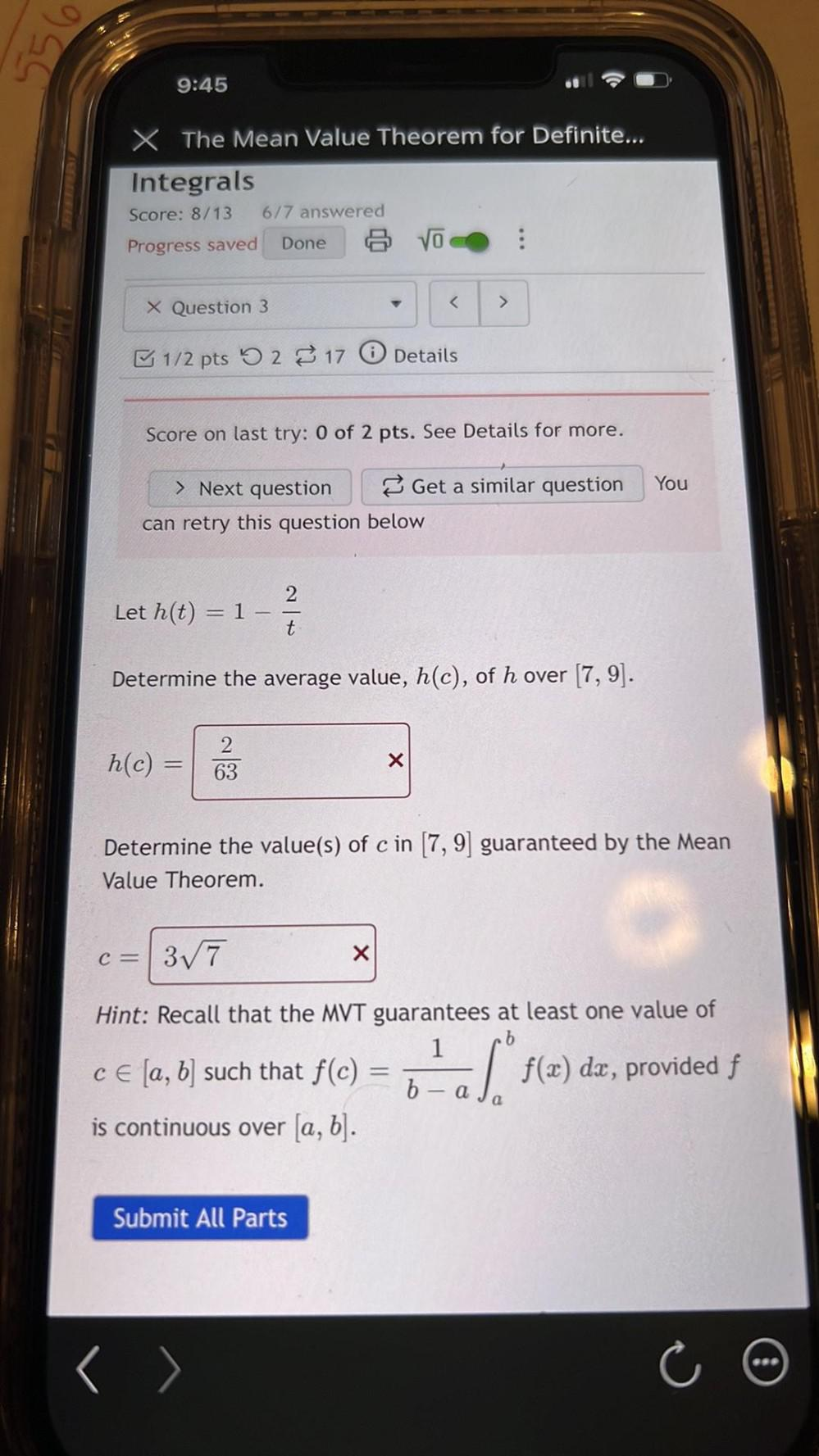Question:

# 554 9:45 X The Mean Value Theorem for Definite... Integrals Score: 8/13 6/7 answered Progress saved Done VO : X Question 3 < > 1554 9:45 X The Mean Value Theorem for Definite... Integrals Score: 8/13 6/7 answered Progress saved Done VO : X Question 3 < > 1/2 pts 5 2 17 0 Details Score on last try: 0 of 2 pts. See Details for more. You > Next question Get a similar question can retry this question below 2 Let h(t) = 1 t Determine the average value, h(c), of h over (7,9). h(c) = 2 63 x Determine the value(s) of c in (7,9) guaranteed by the Mean Value Theorem. C= 37 X Hint: Recall that the MVT guarantees at least one value of b 1 ce [a, b] such that f(c) f(x) dx, provided f x b is continuous over [a, b]. ac) = 6-a 1. a Submit All Parts < >